# An integrated neural network, GoogLeNet

Time：2019-10-23

### 1. Introduction

We’ve already talked about AlexNet, so let’s look at GoogLeNet. GoogLeNet won the first prize in the 2014 ImageNet challenge (ILSVRC14). So how does GoogLeNet improve network performance?
Generally speaking, the most direct way to improve the network performance is to increase the depth and width of the network. Depth refers to the number of network layers and width to the number of neurons. But this approach has the following problems:
(1) with too many parameters, it is easy to generate overfitting if the training data set is limited;
(2) the larger the network and the more parameters, the greater the computational complexity, and it is difficult to apply;
(3) the deeper the network is, the more likely gradient dispersion problem will occur (the further the gradient is, the easier it is to disappear), and it is difficult to optimize the model.
The way to solve these problems is, of course, to increase the depth and width of the network while reducing the parameters. However, in terms of implementation, the actual calculation amount will not be improved substantially after the full connection becomes sparse connection, because most of the hardware is optimized for the calculation of dense matrix. Although the data amount of sparse matrix is small, it is difficult to reduce the calculation time.
Then, is there a method that can not only maintain the sparsity of the network structure, but also take advantage of the high computational performance of the dense matrix? Show that a large number of literature can be sparse matrix clustering for relatively dense sub-matrix to improve computing performance, like the human brain is can be repeated as neurons accumulation, therefore, GoogLeNet team Inception network structure, is to construct a “basic neurons” structure, to build a sparse, the network structure of high computing performance.

### 2. Network structure characteristics

#### [](#0iGxl)2.1 1×1 Convolution

The primary purpose of 1×1 convolution is to reduce dimensions and also to modify linear activation (ReLU). In GoogLeNet, 1×1 convolution is used as a dimensionality reduction module to reduce computation. By reducing computing bottlenecks, you can increase depth and width.
Let’s explain with a small example:
Suppose we need to perform 5×5 convolution without using 1×1 convolution, as follows: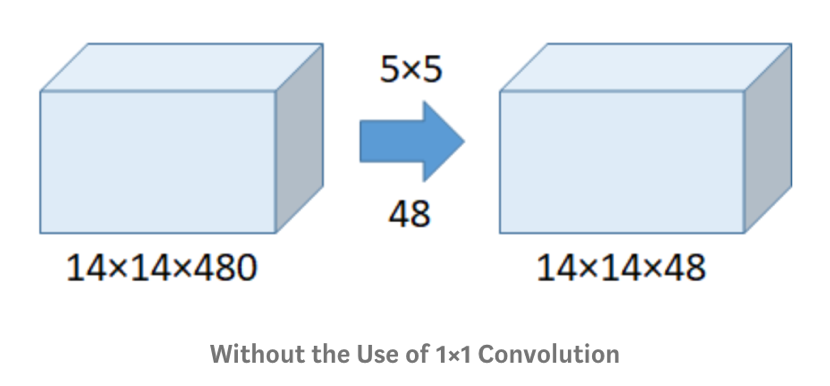Number of calculationsUse 1×1 convolution: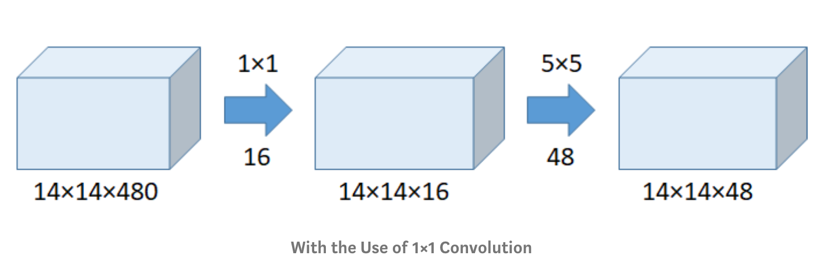The number of convolution operations5 times 5 convolution operationsTotal number of calculationsIs far less than#### [](#lvZ2c)2.2 Inception Module

The original Inception module (initial version, no 1×1 convolution) is as follows: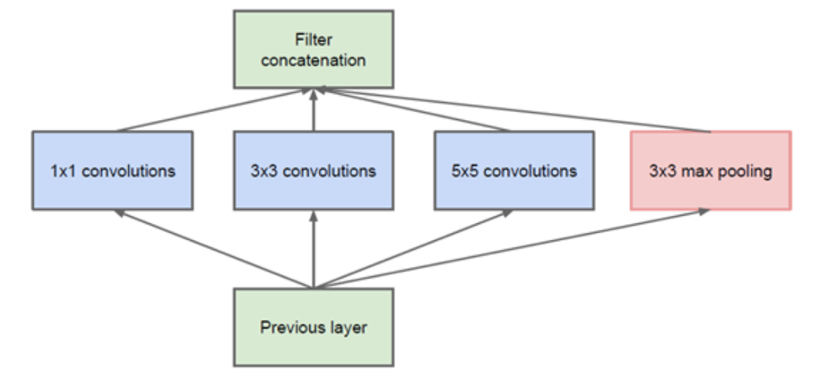In this structure, convolution (1×1, 3×3, 5×5) and pooling operation (3×3) commonly used in CNN are stacked together (convolution and pooling have the same size, and channels are added), which on the one hand increases the width of the network, and on the other hand also increases the adaptability of the network to scale.
The network in the network convolution layer can extract every detail of the input, and the 5×5 filter can also cover most of the input of the receiving layer. A pooling operation can also be performed to reduce the size of the space and reduce overfitting. On top of these layers, a ReLU operation is performed after each convolutional layer to increase the non-linear characteristics of the network.
The Inception of the original version, however, all of the convolution kernels in a layer of all output up to do the calculations required to the 5 x5 convolution kernels quantity is big, caused the thickness of the characteristic diagram is very big, in order to avoid this kind of situation, before 3 x3, 5 x5 before and after Max pooling plus 1 x1 convolution kernels, respectively to have played an important role to reduce thickness of the characteristics, it formed the Inception v1 network structure, as shown in the figure below: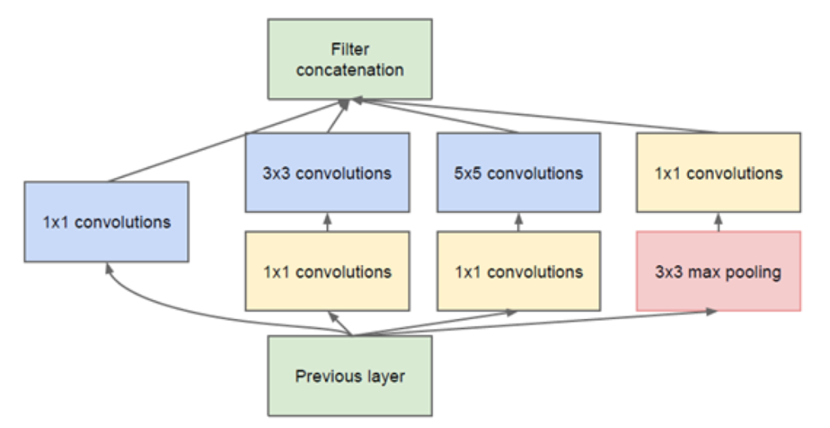#### [](#Evqy7)2.3 global average pooling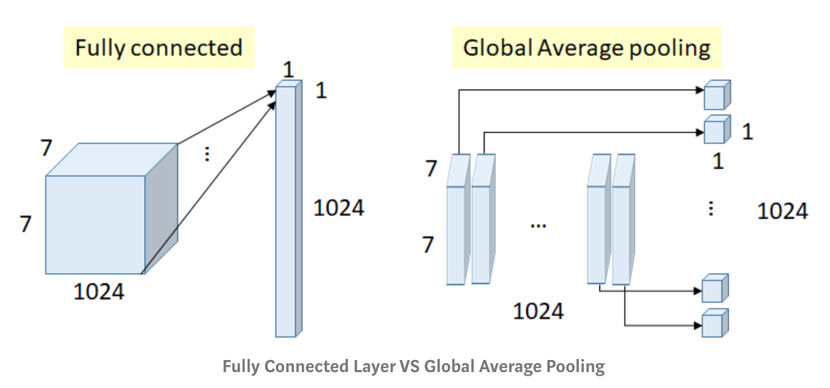Previously full connection (FC) layers were used at the end of the network, such as in AlexNet where all inputs are connected to each output, the number of weightsIn GoogLeNet, by mapping each feature from 7×7 to 1×1 average, the global average pool is almost used at the end of the network, as shown in the figure above, the number of weights. The authors found that the migration from FC layer to average pool improved the accuracy of top-1 by about 0.6%. This also helps to reduce the occurrence of overfitting.

#### [](#aLRgZ)2.4 auxiliary classifier

Softmax branch is introduced in the middle of the network. These branches are auxiliary classifiers, which are composed of 5×5 Average, Pooling (Stride 3), 1×1 Conv (128 filters), 1024 FC, 1000 FC and softmax. They are only used in training, not testing. Losses are weighted at 0.3 to the total loss. Used to combat the gradient vanishing problem, and to provide regularization.

### 3. [] (# uCltU) GoogLeNet model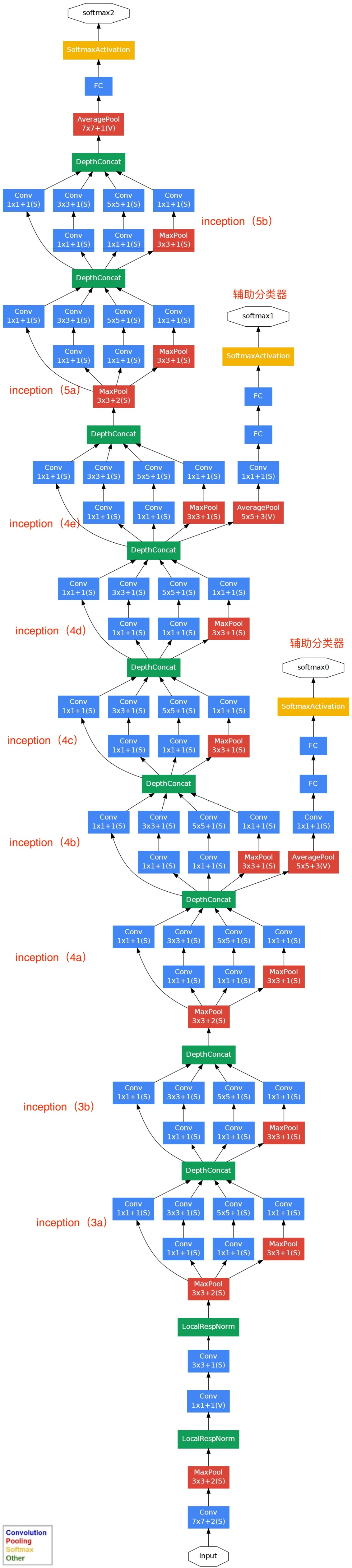The above figure is illustrated as follows:
(2) in the end, the Network adopts average pooling instead of the full connection layer. This idea comes from NIN (Network in Network), which has been proved to improve the accuracy rate by 0.6%. However, in fact, a full connection layer was added in the end, mainly for the convenience of flexible adjustment of the output;
(3) although the full connection is removed, Dropout is still used in the network;
(4) in order to avoid the gradient disappearing, the network added two additional auxiliary softmax for the forward conduction gradient (auxiliary classifier). The auxiliary classifier USES the output of one layer in the middle as classification and adds a smaller weight (0.3) to the final classification result, which is equivalent to model fusion, adding back propagation gradient signal to the network and providing additional regularization, which is beneficial to the training of the whole network. During the actual test, these two extra softmax will be removed.
Details of the network structure of GoogLeNet: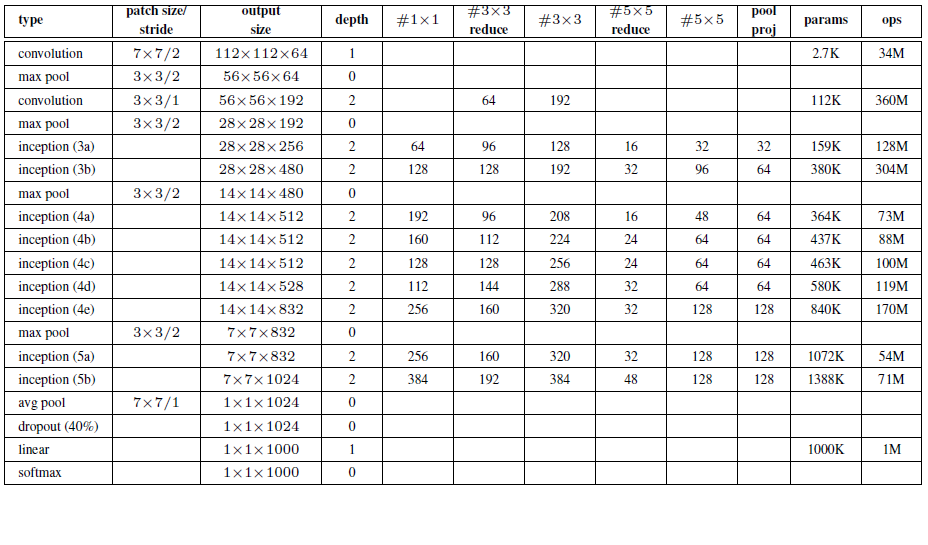Note: “#3×3 reduce” and “#5×5 reduce” in the above table represent the number of convolution operations of 1×1 before 3×3 and 5×5.
The list of network structure of GoogLeNet is as follows:
0, input
The original input image is 224x224x3, and it has been preprocessed with zero mean (subtracting the mean value from each pixel of the image).
1. The first layer (convolution layer)
Use 7×7 convolution kernel (slide step 2, padding 3), 64 channels, output 112x112x64, and perform ReLU operation after convolution
After the Max pooling of 3×3 (step length is 2), the output is (112-3 +1)/2)+1=56, namely 56x56x64, and then ReLU operation is conducted
3. The second layer (convolution layer)
Use the convolution kernel of 3×3 (slide step size is 1, padding is 1), 192 channel, output is 56x56x192, and perform ReLU operation after convolution
After the Max pooling of 3×3 (step length is 2), the output is ((56-3 +1)/2)+1=28, that is, 28x28x192, and then ReLU operation
3a, level 3 (Inception 3a)
It is divided into four branches and processed by convolution kernel of different scales
(1) 64 convolution nuclei of 1×1, and then ReLU, output 28x28x64
(2) 96 convolution kernels of 1×1, as the dimensionality reduction before 3×3 convolution kernels, become 28x28x96, then perform ReLU calculation, and then perform 128 convolution of 3×3 (padding is 1), and output 28x28x128
(3) 16 convolution kernels of 1×1, as the dimensionality reduction before 5×5 convolution kernels, become 28x28x16. After ReLU calculation, 32 convolution kernels of 5×5 (padding is 2) are carried out and output 28x28x32
(4) the pool layer, using the core of 3×3 (padding is 1), outputs 28x28x192, and then carries out the convolution of 32 1×1, outputs 28x28x32.
Connect the four results, parallel the third dimension of the output results of these four parts, namely 64+128+32+32=256, and finally output 28x28x256
3b, layer 3 (Inception 3b)
(1) 128 convolution nuclei of 1×1, and then ReLU outputs 28x28x128
(2) 128 convolution kernels of 1×1, as the dimensionality reduction before 3×3 convolution kernels, become 28x28x128, carry out ReLU, and then carry out 192 convolution of 3×3 (padding is 1), and output 28x28x192
(3) 32 convolution kernels of 1×1, as the dimensionality reduction before 5×5 convolution kernels, become 28x28x32. After ReLU calculation, 96 convolution kernels of 5×5 (padding is 2) are carried out, and the output is 28x28x96
(4) the pool layer, using the core of 3×3 (padding is 1), outputs 28x28x256, and then carries out the convolution of 64 1×1, outputs 28x28x64.
Connect the four results and parallel the third dimension of the output results of these four parts, that is, 128+192+96+64=480. The final output output is 28x28x480
The fourth floor (4a,4b,4c,4d,4e), the fifth floor (5a,5b)… , similar to 3a and 3b, are not repeated here.

### [](#95aCH)4. Summary and outlook

Currently, 17 Flower projects based on the GoogLeNet model can be found in the Mo platform. If you encounter difficulties or find our mistakes in the process of learning, you can contact us through Mo platform or WeChat official account MomodelAI.
The project source address: https://momodel.cn/explore/5d258bfa1afd942ff7b1f521? Type = app

• Inception modules are proposed and optimized
• Cancel full connection layer
• An auxiliary classifier is used to accelerate the convergence of the network

### [](#U43ym)5. References

Paper: https://arxiv.org/abs/1409.4842
Blog: https://blog.csdn.net/Quincuntial/article/details/76457409

Data set: http://www.robots.ox.ac.uk/~vgg/data/flowers/17/

Blog: https://my.oschina.net/u/876354/blog/1637819

Mo(web site:momodel.cn) is one that supports PythonArtificial intelligence online modeling platform, which helps you quickly develop, train, and deploy models.

Mo ai clubIt is initiated by the r&d and product design team of the website and is committed to lowering the threshold of artificial intelligence development and use. The team has experience in big data processing, analysis, visualization and data modeling, has undertaken multi-domain intelligent projects, and has full design and development capabilities from the bottom to the front end. The main research direction is big data management analysis and artificial intelligence technology to promote data-driven scientific research.

At present, the club holds an offline technology salon themed by machine learning in hangzhou every Saturday, and conducts paper sharing and academic exchange from time to time. Hope to gather friends from all walks of life interested in artificial intelligence, continue to exchange and grow together, promote the democratization of artificial intelligence, the application of popularization.## Query SAP multiple database table sizes

Query SAP multiple database table sizes https://www.cnblogs.com/ken-yu/p/12973009.html Item code db02 Here are two approaches, In the first graphical interface, the results of the query data table are displayed in MB, and only one table can be queried at a time. SPACE—Segments—Detailed Analysis—Detailed Analysis  In the pop-up window segment / object, enter the name of the […]